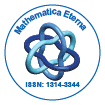Mathematica EternaOpen Access

ISSN: 1314-3344+32-466902147

Abstract

On the oscillatory behavior of solutions of a class of nonlinear fractional difference equations

Qi GE, Chengmin HOU

In this paper, we study the oscillatory behavior of solutions of a class of Caputo fractional difference equations. Some new criteria for the oscillation of fractional difference equations is established.

Top